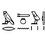# Too many 3s

A number written in base $10$ is a string of $3^{2013}$ digit $3$s. No other digit appears. Find the highest power of $3$ that divides this number.

Source: BMO, November 2013Note by Mark Hennings
6 years, 9 months ago

This discussion board is a place to discuss our Daily Challenges and the math and science related to those challenges. Explanations are more than just a solution — they should explain the steps and thinking strategies that you used to obtain the solution. Comments should further the discussion of math and science.

When posting on Brilliant:

• Use the emojis to react to an explanation, whether you're congratulating a job well done , or just really confused .
• Ask specific questions about the challenge or the steps in somebody's explanation. Well-posed questions can add a lot to the discussion, but posting "I don't understand!" doesn't help anyone.
• Try to contribute something new to the discussion, whether it is an extension, generalization or other idea related to the challenge.

MarkdownAppears as
*italics* or _italics_ italics
**bold** or __bold__ bold
- bulleted- list
• bulleted
• list
1. numbered2. list
1. numbered
2. list
Note: you must add a full line of space before and after lists for them to show up correctly
paragraph 1paragraph 2

paragraph 1

paragraph 2

[example link](https://brilliant.org)example link
> This is a quote
This is a quote
    # I indented these lines
# 4 spaces, and now they show
# up as a code block.

print "hello world"
# I indented these lines
# 4 spaces, and now they show
# up as a code block.

print "hello world"
MathAppears as
Remember to wrap math in $$ ... $$ or $ ... $ to ensure proper formatting.
2 \times 3 $2 \times 3$
2^{34} $2^{34}$
a_{i-1} $a_{i-1}$
\frac{2}{3} $\frac{2}{3}$
\sqrt{2} $\sqrt{2}$
\sum_{i=1}^3 $\sum_{i=1}^3$
\sin \theta $\sin \theta$
\boxed{123} $\boxed{123}$

Sort by:

The expression equals to $33 \ldots 3 = \frac {1}{3} (99 \ldots 9 ) = \frac {1}{3} (10^{3^{2013}} - 1)$

We want to evaluate

$v_p ( \frac {1}{3} (10^{3^{2013}} - 1)) = -1 + v_p (10^{3^{2013}} - 1)$

With $p = 3, n = 3^{2013}, x = 10, y = -1$, we have

$-1 + v_3 (10 + (-1)) + v_3 (3^{2013} ) = -1 + 2 + 2013 = 2014$

Hope I'm right, I'm still very new to Lifting The Exponent

Side question: How do you set on LaTeX to show that underneath $33 \ldots 3$, it has $3^{2013}$ digits?

- 6 years, 9 months ago

\underbrace{333...3}_{3^{2013}\ digits} gives $\underbrace{333...3}_{3^{2013}\ digits}$

EDIT: Also, 2014 is correct. I sat this exam last week.

- 6 years, 9 months ago

\text{digits} gives $\text{digits}$, which allows you to have 'words' within a mathematical statement, so that it does't appear in italic.

\underbrace{333 \ldots 3} _ {3^{2013} \text{ digits} } gives $\underbrace{333 \ldots 3} _ {3^{2013} \text{ digits} }$.

Staff - 6 years, 9 months ago

$\underbrace{ \text{THANKYOUTHANKYOU} \ldots \text{THANKYOU}} _ { \text{ gazillion times} }$

- 6 years, 9 months ago

\mbox{digits} works just as well as \text{digits}, but is perhaps less memorable! The one to be careful with is \mathrm. If you \mathrm{use that one}, you $\mathrm{lose the spaces,}$ since LaTeX ignores all spacing in mathematical typesetting, and \mathrm just forces an upright font, without switching out of Maths mode.

- 6 years, 9 months ago

$\mbox{Thank you} \space \text{Sir Mark Hennings }, \mathrm{You're A \omega esome}!$

- 6 years, 9 months ago

You don't have to appeal to LTE. If $N(x,n)$ is the number obtained by concatenating the number $x$ a total of $n$ times, so that $N(12,3) = 121212$, then $N(x,3) \; = \; (10^{2k} + 10^k + 1)x$ for any $k$-digit number $x$. Since the sum of the digits of $10^{2k} + 10^k + 1$ is $3$, it contains exactly one factor of $3$, and so the exponent of $3$ in $N(x,3)$ is one more than the exponent of $3$ in $x$. Since $N(3,3^{n+1}) = N(N(3,3^n),3)$ an inductive argument finishes things off nicely.

- 6 years, 9 months ago

Another way of writing this down. Let $x_n$ represent the number that when written in decimal representation is a string of $3^n$ digits 1s only. We can see that $x_n=(10^{2(3^{n-1})}+10^{3^{n-1}}+1)x_{n-1}.$ Therefore, it can be proved that $x_n=\prod_{k=0}^{n-1}(10^{2(3^{k-1})}+10^{3^{k-1}}+1)$. Each factor of this product is divisible by $3$ but not by $3^2.$ Therefore the highest power of 3 that is a factor of $x_n$ is $3^n.$. The number of the question is equal to $3x_{2013}.$ That is why the highest power of 3 that divides this number is $3^{2014}.$

- 4 years, 4 months ago

The ans is $3^{2014}$

The divisibility theory states that for any number to be divisible for 3, the sum of its digits must be divisible by 3. Similarly, for any number to be divisible for $3^{2}$, the sum of its digits must be divisible by $3^{2}$ and so on.

for any number to be divisible for $3^{n}$, the sum of its digits must be divisible by $3^{n}$

for divisibility by $3^{3}$, Consider any random number.

Let it be 2457396234. Sum of its digits is 2+4+5+7+3+9+6+2+3+4=45 Hence it is not divisible by 27 as 45 is not divisible by 27. But is is divisible by 9, as 45 is divisible by 9.

It leaves a remainder of 9 when divided by 27 with a quotient of 91014675.

It leaves a remainder of 0 when divided by 9 with a quotient of 273044026.

So the sum of the digits of the number in the question is $3* 3^{2013} = 3^{2014}$

hence it is divisible by $3^{2014}$.

- 5 years, 2 months ago

The case for n=1 and 2 are true, but it is not true for n = 3. For instance, 27 is obviously divisible by 27 but 2+7=9 is not divisible by 27. That is why your answer is wrong.

- 4 years, 8 months ago